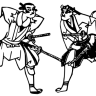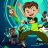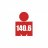# Big Days Indicator for ThinkorSwim

E

#### ext99k

##### Member
This is a new custom indicator the great @Welkin created for me, that counts the number of bars (used on daily aggregation) until the user defined daily change from the previous bar has occurred. I posted it because it's great and I think everyone would appreciate it, and it's always nice to pay it forward so thanks again to @Welkin for the great work.

For example, if 5% daily move is set, starting from the beginning of the period, it counts the number of days until the condition is true, then, it resets and begins to count from zero again until the condition is true again (this could be set in the options).

Green counts the number of days until the daily price change exceeds the benchmark
Red counts the number of days it took for the daily price change to be below the benchmark
Yellow indicates the total number of true events (for both price exceeding and falling below the benchmark)

In the options, the "postest" and "negtest" lines simply show 1 or 0 depending if the event is true. You could hide these by default when using TOS on your PC, and show them on mobile, given mobile app limitations, that's the only way you could get the indicator to work on mobile.

The study is great for identifying clusters of volatility.

You'll likely notice once a condition is triggered for a relatively high daily price change benchmark like 5%, there tends to be a continuation of volatility the very short-run.Code:
``````#[email protected]

declare lower;
def NA = Double.NaN;

input showTargetEvents = yes;
input showPosEvents = yes;
input showNegEvents = yes;
input UseAnchor = no;
input anchorDate = 20190805;
input setPercentageMove = 5;
input AlertCriteria = {default Both, Positive, Negative};

def YYYYMMDD = GetYYYYMMDD();
def conf = YYYYMMDD >= anchorDate;
def agg = GetAggregationPeriod();
def dayAggCheck = agg == AggregationPeriod.DAY;
AddLabel(!dayAggCheck, "Set Aggregation to Daily", Color.Yellow);

def prcnt = setPercentageMove/100;
def c = close;
def change = (((c / c)-1)*100);

#These test plots are enabled for use in custom scan condition wizard
plot targettest = AbsValue(change) >= setPercentageMove;
plot postest = change > setPercentageMove;
plot negtest = change < -setPercentageMove;
targettest.Hide();
postest.Hide();
negtest.Hide();

def Criteria;
case Both:
Criteria = targettest;
case Positive:
Criteria = postest;
case Negative:
Criteria = negtest;
}

def poschange = c + (c * prcnt);
def negchange = c - (c * prcnt);
def targetcount = if UseAnchor and conf and targettest then targetcount + 1  else if !UseAnchor and targettest then targetcount + 1 else targetcount;
def poscount = if UseAnchor and conf and postest then poscount + 1  else if !UseAnchor and postest then poscount + 1 else poscount;
def negcount = if UseAnchor and conf and negtest then negcount + 1  else if !UseAnchor and negtest then negcount + 1 else negcount;
def prevtargetval = if targetcount == 1 then targetcount else NA;
def prevposval = if poscount ==1 then poscount else NA;
def prevnegval = if negcount ==1 then negcount else NA;
def sincelasttarget = if UseAnchor and conf and targettest and targettest then 1 else if UseAnchor and conf and targettest and !targettest then 2 else if UseAnchor and conf and !targettest then sincelasttarget + 1 else if !UseAnchor and targettest and targettest then 1 else if !UseAnchor and targettest and !targettest then 2 else if !UseAnchor and !targettest then sincelasttarget + 1 else sincelasttarget;
def sincelastpos = if UseAnchor and conf and postest and postest then 1 else if UseAnchor and conf and postest and !postest then 2 else if UseAnchor and conf and !postest then sincelastpos + 1 else if !UseAnchor and postest and postest then 1 else if !UseAnchor and postest and !postest then 2 else if !UseAnchor and !postest then sincelastpos + 1 else sincelastpos;
def sincelastneg = if UseAnchor and conf and negtest and negtest then 1 else if UseAnchor and conf and negtest and !negtest then 2 else if UseAnchor and conf and !negtest then sincelastneg + 1 else if !UseAnchor and negtest and negtest then 1 else if !UseAnchor and negtest and !negtest then 2 else if !UseAnchor and !negtest then sincelastneg + 1 else sincelastneg;
def targetvalstore = if targettest then sincelasttarget else NA;
def posvalstore = if postest then sincelastpos else NA;
def negvalstore = if negtest then sincelastneg else NA;
def sumtarget = if UseAnchor and conf and targettest then sumtarget + sincelasttarget else if !UseAnchor and targettest then sumtarget + sincelasttarget else sumtarget;
def sumpos = if UseAnchor and conf and postest then sumpos + sincelastpos else if !UseAnchor and postest then sumpos + sincelastpos else sumpos;
def sumneg = if UseAnchor and conf and negtest then sumneg + sincelastneg else if !UseAnchor and negtest then sumneg + sincelastneg else sumneg;

#These avgs are set as plots for use in custom scan condition wizard
plot targetavg = sumtarget/targetcount;
plot posavg = sumpos / poscount;
plot negavg = sumneg / negcount;
targetavg.Hide();
targetavg.HideTitle();
posavg.Hide();
posavg.HideTitle();
negavg.Hide();
negavg.HideTitle();

#PLOTS
plot posline = setPercentageMove;
plot negline = -setPercentageMove;
plot targetChange = if showTargetEvents and targettest then 0 else NA;
plot pos = if showPosEvents and change > 0 then change else NA;
plot neg = if showNegEvents and change < 0 then change else NA;

#CHART BUBBLES
AddChartBubble(showTargetEvents and targettest, targetChange, sincelasttarget, Color.YELLOW, yes);
AddChartBubble(showPosEvents and postest, posline, sincelastpos, Color.GREEN, yes);
AddChartBubble(showNegEvents and negtest, negline, sincelastneg, Color.RED, no);

#LABELS
AddLabel(1,"Change "+Round(change,2)+"%",if postest then Color.GREEN else if negtest then Color.RED else Color.GRAY);
AddLabel(1,"Prev "+Round(change,2)+"%",if postest then Color.GREEN else if negtest then Color.RED else Color.GRAY);
AddLabel(showNegEvents, "TotalNegEvents | "+ negcount, Color.RED);
AddLabel(showTargetEvents, "Days Since Last : "+ sincelasttarget+" | Avg : "+ Round(targetavg,0) +" | High : " + HighestAll(targetvalstore)+ " | Low : "+ LowestAll(targetvalstore) , Color.YELLOW);
AddLabel(showPosEvents, "Days Since Last : "+ sincelastpos+" | Avg : "+ Round(posavg,0) + " | High : " + HighestAll(posvalstore)+ " | Low : "+ LowestAll(posvalstore) , Color.GREEN);
AddLabel(showNegEvents, "Days Since Last : "+ sincelastneg+" | Avg : "+ Round(negavg,0) + " | High : " + HighestAll(negvalstore)+ " | Low : "+ LowestAll(negvalstore) , Color.RED);

#FORMAT
targettest.SetDefaultColor(Color.YELLOW);
postest.SetDefaultColor(Color.GREEN);
negtest.SetDefaultColor(Color.RED);
pos.AssignValueColor(if !postest and change > 0 then Color.DARK_GREEN else if postest then Color.GREEN else Color.GRAY);
neg.AssignValueColor(if !negtest and change < 0 then Color.DARK_RED else if negtest then Color.RED else Color.GRAY);
pos.SetPaintingStrategy(PaintingStrategy.SQUARED_HISTOGRAM);
neg.SetPaintingStrategy(PaintingStrategy.SQUARED_HISTOGRAM);
pos.SetHiding(!showPosEvents);
neg.SetHiding(!showNegEvents);
posline.SetDefaultColor(Color.DARK_GRAY);
negline.SetDefaultColor(Color.DARK_GRAY);
targetChange.SetHiding(!showTargetEvents);
targetChange.SetDefaultColor(Color.YELLOW);
targetChange.SetPaintingStrategy(PaintingStrategy.POINTS);``````

Last edited:
••Big Bar Breakout Indicator for ThinkorSwim Indicators 9Long Term Indicators: Big Hand and Coppock Indicator for ThinkorSwim Indicators 40x% breakout in 20 days and above 10 & 20 EMA? Questions 1